# sfepy.terms.terms_shells module¶

Terms implementing shell elements.

class sfepy.terms.terms_shells.Shell10XTerm(name, arg_str, integral, region, **kwargs)[source]

The shell10x element term based on the Reissner-Mindlin theory , , corresponding to a shell of thickness.

The term requires a custom 3D quadrature, where thecomponents of quadrature point coordinates are transformed fromto, and the quadrature weights are multiplied by. The variablesandhave to use Shell10XField and have six components. The reference element mapping is implemented by Shell10XMapping. The term does not implement the piezo-electric components of the shell10x element yet.

The term has to be used with quadrilateral cells in 3D and should behave as the linear elastic term, but with fewer degrees of freedom for the same accuracy for shell-like structures. The shell has six degrees of freedom in each of the four nodes: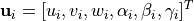,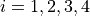. The strain and stress vectors are calculated in a local (co-rotational) coordinate system given by basis vectors,and. It holds that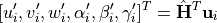where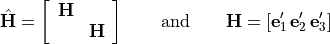is a nodal DOF transformation matrix.

The local displacements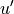,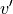and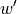at any point in the layer characterized by the isoparametric coordinates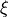,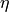and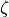(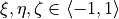) are interpolated from the nodal displacement and rotation values (i.e. both membrane and bending components) using standard isoparametric approximation functions for a quadrilateral, hence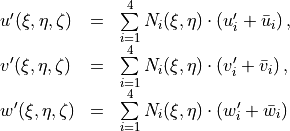where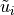,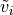andare the bending components of displacements calculated from displacements due to rotations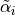and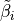about local nodal axes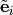as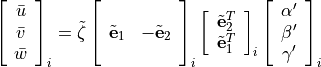where. The local nodal axesare constructed in order to describe the behavior of warped (non-planar) elements adequately.

The term employs three shell element enhancements:

• DSG method

• EAS method

• drilling rotations lock (parameter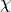- a good value is about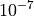)

For detailed theoretical information see the references.

1

Zemčík, R., Rolfes, R., Rose, M. and Tessmer, J. (2006),

High-Performance 4-Node Shell Element with Piezoelectric Coupling Mechanics of Advanced Materials and Structures Vol. 13, Iss. 5, doi:10.1080/15376490600777657

2

Zemčík, R., Rolfes, R., Rose, M. and Teßmer, J. (2007),

High-performance four-node shell element with piezoelectric coupling for the analysis of smart laminated structures. Int. J. Numer. Meth. Engng., 70: 934–961. doi:10.1002/nme.1909

Definition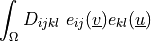Call signature

 dw_shell10x (material_d, material_drill, virtual, state)
Arguments
• material_d :• material_drill :• virtual :• state :arg_shapes = {'material_d': '6, 6', 'material_drill': '.: 1', 'state': 6, 'virtual': (6, 'state')}
arg_types = ('material_d', 'material_drill', 'virtual', 'state')
static function(out, mtx_k, el_u, fmode)[source]
geometries = ['3_2_4']
get_fargs(mtx_d, drill, virtual, state, mode=None, term_mode=None, diff_var=None, **kwargs)[source]
get_physical_qps()[source]

Get physical quadrature points corresponding to the term region and integral.

integration = 'custom'
name = 'dw_shell10x'
poly_space_base = 'shell10x'
set_integral(integral)[source]

Set the term integral.